### Home > AC > Chapter 14 > Lesson 14.2.1.8 > Problem2-112

2-112.
1. Find a rule for each sequence. Homework Help ✎

1. t(n)=number of tiles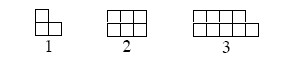2. n

t(n)

1

7

2

4

3

1

3. n

t(n)

5

−20

6

−10

7

−5

4. Year

Cost

2

$2000 3$6000

4

\$18000

5.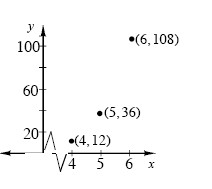6.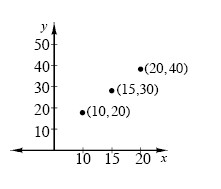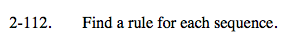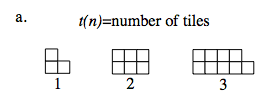t(n) = 3n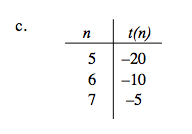$\textit{t}(\textit{n})=-20\left(\frac{1}{2}\right)^{\textit{n}-5}\text{ or }\textit{t}(\textit{n})=-640\left(\frac{1}{2}\right)^\textit{n}$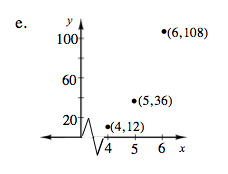$\textit{t}(\textit{n})=12(3)^{\textit{n}-4}\text{ or }\textit{t}(\textit{n})=\frac{4}{27}(3)^\textit{n}$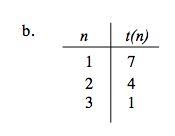t(n) = −3n + 10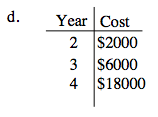See part (c).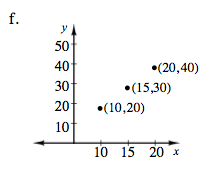See part (e).